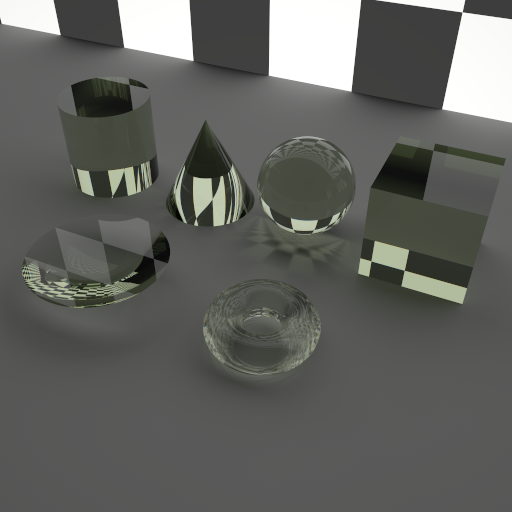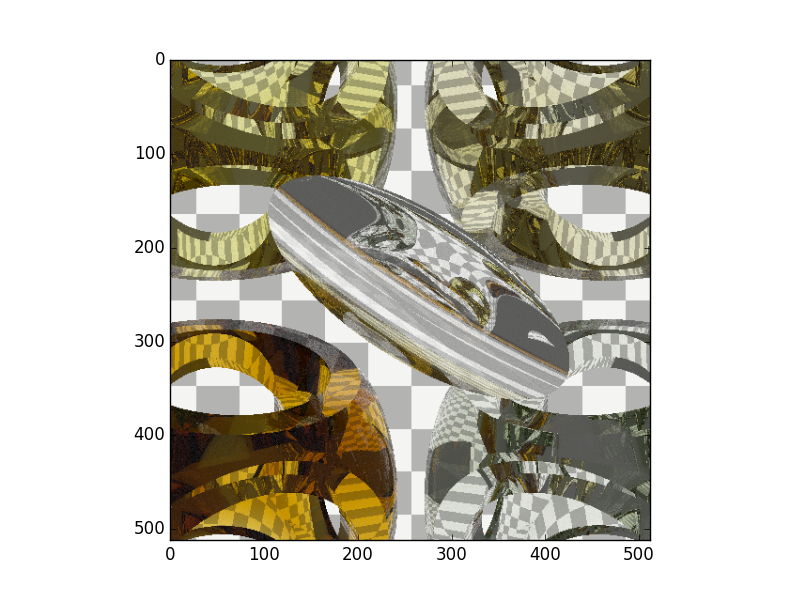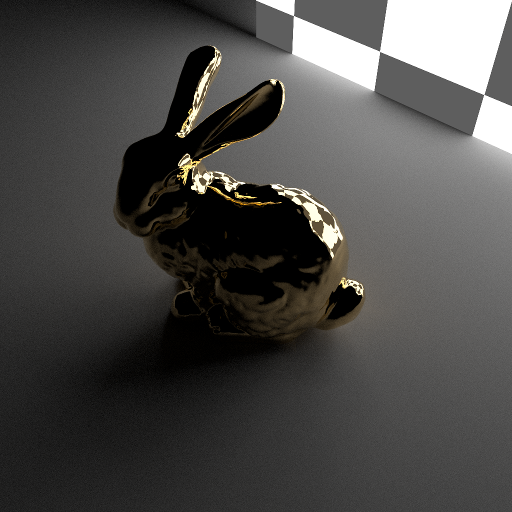# 7. Primitives¶

The raysect primitives: sphere; box; cylinder; and cone.## 7.1. Geometric Primitives¶

### 7.1.1. Sphere¶

class raysect.primitive.Sphere

A sphere primitive.

The sphere is centered at the origin of the local co-ordinate system.

Parameters
• radius (float) – Radius of the sphere in meters (default = 0.5).

• parent (Node) – Scene-graph parent node or None (default = None).

• transform (AffineMatrix3D) – An AffineMatrix3D defining the local co-ordinate system relative to the scene-graph parent (default = identity matrix).

• material (Material) – A Material object defining the sphere’s material (default = None).

• name (str) – A string specifying a user-friendly name for the sphere (default = “”).

Variables

>>> from raysect.core import translate
>>> from raysect.primitive import Sphere
>>> from raysect.optical import World
>>> from raysect.optical.material import UniformSurfaceEmitter
>>> from raysect.optical.library.spectra.colours import orange
>>>
>>> world = World()
>>>
>>> sphere = Sphere(2.5, parent=world, transform=translate(3, 0, 0),
material=UniformSurfaceEmitter(orange), name="orange sphere")


### 7.1.2. Box¶

class raysect.primitive.Box

A box primitive.

The box is defined by lower and upper points in the local co-ordinate system.

Parameters
• lower (Point3D) – Lower point of the box (default = Point3D(-0.5, -0.5, -0.5)).

• upper (Point3D) – Upper point of the box (default = Point3D(0.5, 0.5, 0.5)).

• parent (Node) – Scene-graph parent node or None (default = None).

• transform (AffineMatrix3D) – An AffineMatrix3D defining the local co-ordinate system relative to the scene-graph parent (default = identity matrix).

• material (Material) – A Material object defining the box’s material (default = None).

• name (str) – A string specifying a user-friendly name for the box (default = “”).

>>> from raysect.core import Point3D, translate
>>> from raysect.primitive import Box
>>> from raysect.optical import World
>>> from raysect.optical.material import UniformSurfaceEmitter
>>> from raysect.optical.library.spectra.colours import red
>>>
>>> world = World()
>>>
>>> cube = Box(Point3D(0,0,0), Point3D(1,1,1), parent=world, transform=translate(0, 1, 0),
material=UniformSurfaceEmitter(red), name="red cube")


### 7.1.3. Cylinder¶

class raysect.primitive.Cylinder

A cylinder primitive.

The cylinder is defined by a radius and height. It lies along the z-axis and extends over the z range [0, height]. The ends of the cylinder are capped with disks forming a closed surface.

Parameters
• radius (float) – Radius of the cylinder in meters (default = 0.5).

• height (float) – Height of the cylinder in meters (default = 1.0).

• parent (Node) – Scene-graph parent node or None (default = None).

• transform (AffineMatrix3D) – An AffineMatrix3D defining the local co-ordinate system relative to the scene-graph parent (default = identity matrix).

• material (Material) – A Material object defining the cylinder’s material (default = None).

• name (str) – A string specifying a user-friendly name for the cylinder (default = “”).

>>> from raysect.core import translate
>>> from raysect.primitive import Cylinder
>>> from raysect.optical import World
>>> from raysect.optical.material import UniformSurfaceEmitter
>>> from raysect.optical.library.spectra.colours import blue
>>>
>>> world = World()
>>>
>>> cylinder = Cylinder(0.5, 2.0, parent=world, transform=translate(0, 0, 1),
material=UniformSurfaceEmitter(blue), name="blue cylinder")


### 7.1.4. Cone¶

class raysect.primitive.Cone

A cone primitive.

The cone is defined by a radius and height. It lies along the z-axis and extends over the z range [0, height]. The tip of the cone lies at z = height. The base of the cone sits on the x-y plane and is capped with a disk, forming a closed surface.

Parameters
• radius (float) – Radius of the cone in meters in x-y plane (default = 0.5).

• height (float) – Height of the cone in meters (default = 1.0).

• parent (Node) – Scene-graph parent node or None (default = None).

• transform (AffineMatrix3D) – An AffineMatrix3D defining the local co-ordinate system relative to the scene-graph parent (default = identity matrix).

• material (Material) – A Material object defining the cone’s material (default = None).

• name (str) – A string specifying a user-friendly name for the cone (default = “”).

>>> from raysect.core import translate
>>> from raysect.primitive import Box
>>> from raysect.optical import World
>>> from raysect.optical.material import UniformSurfaceEmitter
>>> from raysect.optical.library.spectra.colours import green
>>>
>>> world = World()
>>>
>>> cone = Cone(0.5, 2.0, parent=world, transform=translate(0, 0, 1),
material=UniformSurfaceEmitter(green), name="green cone")


## 7.2. CSG Operations¶

Operations such as union, substract, intersect on some basic glass primitives:

# Making the lense in the centre
s1 = Sphere(1.0, transform=translate(0, 0, 1.0-0.01))
s2 = Sphere(0.5, transform=translate(0, 0, -0.5+0.01))
Intersect(s1, s2, world, translate(0,0,-3.6)*rotate(50,50,0), glass)

# More complex glass structure
cyl_x = Cylinder(1, 4.2, transform=rotate(90, 0, 0)*translate(0, 0, -2.1))
cyl_y = Cylinder(1, 4.2, transform=rotate(0, 90, 0)*translate(0, 0, -2.1))
cyl_z = Cylinder(1, 4.2, transform=rotate(0, 0, 0)*translate(0, 0, -2.1))
cube = Box(Point3D(-1.5, -1.5, -1.5), Point3D(1.5, 1.5, 1.5))
sphere = Sphere(2.0)

Intersect(sphere, Subtract(cube, Union(Union(cyl_x, cyl_y), cyl_z)), world, translate(-2.1,2.1,2.5)*rotate(30, -20, 0), glass)## 7.3. Meshes¶

It is easiest to import meshes from existing CAD files in either obj or stl with the helper methods.

raysect.primitive.mesh.obj.import_obj(filename, scaling=1.0, **kwargs)

Create a mesh instance from a Wavefront OBJ mesh file (.obj).

Parameters
• filename (str) – Mesh file path.

• scaling (double) – Scale the mesh by this factor (default=1.0).

• kwargs – Accepts optional keyword arguments from the Mesh class.

Return type

Mesh

>>> from raysect.optical import World, translate, rotate, ConstantSF, Sellmeier, Dielectric
>>> from raysect.primitive import import_obj
>>>
>>> world = World()
>>>
>>> diamond = Dielectric(Sellmeier(0.3306, 4.3356, 0.0, 0.1750**2, 0.1060**2, 0.0),
>>>                      ConstantSF(1.0))
>>>
>>> bunny_mesh = import_obj("resources/stanford_bunny.obj", scaling=1, parent=world,
>>>                         transform=translate(0, 0, 0)*rotate(165, 0, 0), material=diamond)

raysect.primitive.mesh.stl.import_stl(filename, scaling=1.0, mode='auto', **kwargs)

Create a mesh instance from a STereoLithography (STL) mesh file (.stl).

Some engineering meshes are exported in different units (mm for example) whereas Raysect units are in m. Applying a scale factor of 0.001 would convert the mesh into m for use in Raysect.

Parameters
• filename (str) – Mesh file path.

• scaling (double) – Scale the mesh by this factor (default=1.0).

• mode (str) – The file format to load: ‘ascii’, ‘binary’, ‘auto’ (default=’auto’).

• kwargs – Accepts optional keyword arguments from the Mesh class.

Return type

Mesh

>>> from raysect.optical import World, translate, rotate, ConstantSF, Sellmeier, Dielectric
>>> from raysect.primitive import import_stl
>>>
>>> world = World()
>>>
>>> diamond = Dielectric(Sellmeier(0.3306, 4.3356, 0.0, 0.1750**2, 0.1060**2, 0.0),
>>>                      ConstantSF(1.0))
>>>
>>> mesh = import_stl("my_mesh.stl", scaling=1, mode='binary', parent=world,
>>>                   transform=translate(0, 0, 0)*rotate(165, 0, 0), material=diamond)


An example:

from raysect.primitive.mesh import import_obj
mesh = import_obj("./resources/stanford_bunny.obj", scaling=1, parent=world,
transform=translate(0, 0, 0)*rotate(165, 0, 0), material=gold)